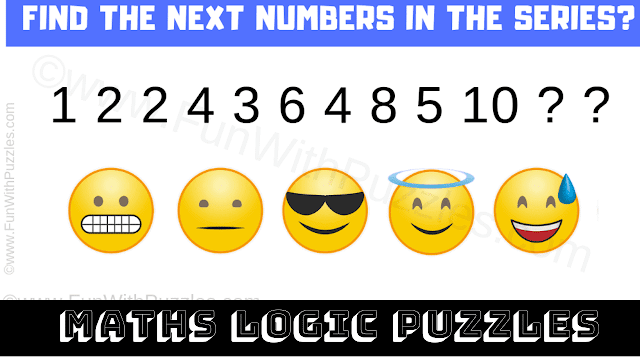This Puzzle Video contains the logical reasoning puzzles to test your brain. In this puzzle video, there are 5 maths logic puzzles. In each of these puzzles, you will see some number equations or a sequence of numbers. Your challenge is to crack the hidden pattern in these number sequences. Once you are able to crack the code, find the value of the missing number which will replace the question mark.
You will get 15 seconds to solve each logical reasoning puzzle. However, if you think that more time is required to solve a particular number equation, then you can pause the video. Write down your answer to the last logic puzzle in the comments.